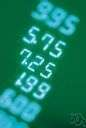algorism

Also found in: Thesaurus, Medical, Financial, Encyclopedia, Wikipedia.

al·go·rism

(ăl′gə-rĭz′əm)
n.
1. The Arabic system of numeration; the decimal system.
2. Computation with Arabic figures.

[Middle English algorisme, from Old French, from Medieval Latin algorismus, after Muhammad ibn-Musa al-Khwarizmi.]

algorism

(ˈælɡəˌrɪzəm)
n
1. (Mathematics) the Arabic or decimal system of counting
2. (Mathematics) the skill of computation using any system of numerals
3. (Mathematics) another name for algorithm
[C13: from Old French algorisme, from Medieval Latin algorismus, from Arabic al-khuwārizmi, from the name of abu-Ja`far Mohammed ibn-Mūsa al-Khuwārizmi, ninth-century Persian mathematician]

al•go•rism

(ˈæl gəˌrɪz əm)

n.
the Arabic system of arithmetical notation (with the figures 1, 2, 3, etc.).
[1200–1250; Middle English augrim, algrim < Middle French < Medieval Latin algorismus < Arabic al the + kh(u)wārizmī (surname of a 9th-century Muslim mathematician) =khwārizm Khiva + suffix of appurtenance]

algorism

1. the Arabic system of numbering.
2. the method of computation with the Arabic flgures 1 through 9, plus the zero; arithmetic.
3. the rule for solving a specific kind of arithmetic problem, as finding an average; algorithm. — algorist, n. — algorismic, adj.
 Noun 1algorism - the Arabic (or decimal) system of numerationdecimal number system, decimal numeration system, decimal system - a positional system of numeration that uses decimal digits and a base of ten 2algorism - computation with Arabic figures  arithmetic - the branch of pure mathematics dealing with the theory of numerical calculations